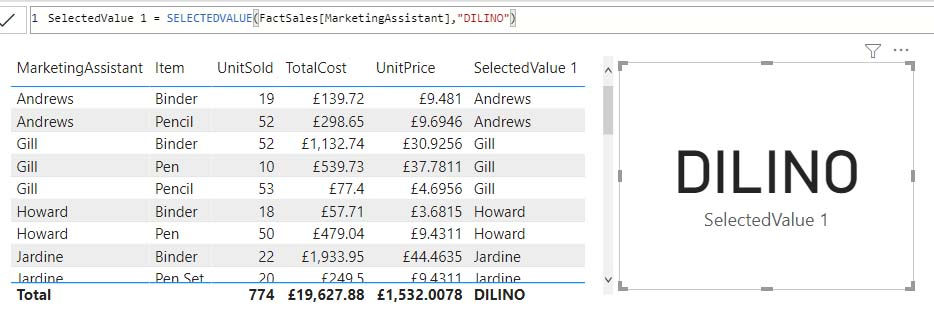top of page
Search
• Marek Vavrovic

# Filter Functions, part two.

Updated: Sep 21, 2021

## CALCULATETABLE

syntax:

CALCULATETABLE(<expression>[, <filter1> [, <filter2> [, …]]])

Every filter argument can be either a filter removal (such as ALL, ALLEXCEPT, ALLNOBLANKROW), a filter restore (ALLSELECTED), or a table expression returning a list of values for one or more columns or for an entire expanded table.

Example 1

with SUMX function

In this measure, CALCULATETABLE returns a filtered table where Item="Pen". SUMX function calculates SUM of all ItemSold for each row.

Total number of pen sold = SUMX( CALCULATETABLE(StoreA,StoreA[Item]="Pen") ,StoreA[ItemsSold])Example 2

with FILTER(ALL()...)

When a filter argument has the form of a predicate with a single column reference, the expression is embedded into a FILTER expression that filters all the values of the referenced column.

Average Male Salary = AVERAGEX( CALCULATETABLE(DimCustomer, FILTER( ALL(DimCustomer), DimCustomer[Gender]="M")) ,DimCustomer[Salary])

this function returns the average male's salary for each row.Example 3

This function calculates the salary for each row where birthdate is not null.## EARLIER

Returns the value in the column prior to the specified number of table scans (default is 1).

syntax

EARLIER(<column>, <number>)

Example 1

adding a column to an existing table

in this example, I calculate the number of transactions in one particular day.Example 2

EARLIER in measure.Example 3

Calculate cumulative total

Earlie RT = CALCULATE( SUM(tblOrders[Quantity]), FILTER(tblOrders,EARLIER(tblOrders[Date],1)>=tblOrders[Date]))## KEEPFILTERS

Modifies how filters are applied while evaluating a CALCULATE or CALCULATETABLE function.

syntax:

KEEPFILTERS(<expression>)

Example 1

Gill sales = CALCULATE(SUM(StoreA[ItemsSold]), KEEPFILTERS(StoreA[Person]="Gill"))Another way how to write this measure is using a combination of FILETER(VALUES(<table>)Example 2

I want to calculate a measure for the Product_A where Quantity > 5

Result = CALCULATE ( SUM ( tblOrders[Quantity] ), KEEPFILTERS ( FILTER ( ALL ( tblOrders[Product], tblOrders[Quantity] ), tblOrders[Product] = "Product_A" ) ), tblOrders[Quantity] > 5 )## SELECTEDVALUE

Returns the value when there’s only one value in the specified column, otherwise returns the alternate result.

syntax:

SELECTEDVALUE(<columnName>[, <alternateResult>])

alternatively you can use

IF(HASONEVALUE(<columnName>),VALUES(<columnName>),<alternateResult>)

works nice with categorical data !